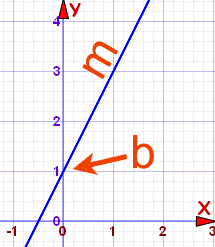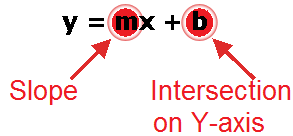# Linear Equation

Linear equations are based on ratio and proportion which we have already discussed in the previous chapter.
·       linear equation is an algebraic equation in which each term is either a constant or the product of a constant and (the first power of) a single variable.

·       Linear equations can have one or more variables.Example:
The monthly income of A & B are in the ratio 6:5 and their expenses are in the ratio 9:7. Saving of A and B are 300 and 500 respectively. Find the income of A.

Income:  A = 6x,    B = 5x
Expense A = 9y,    B = 7y
Saving    A = 300,  B = 500
As given;
6x-9y = 300
5x-7y = 500
After solving these eq. we will get;
X= 800
Y = 500
Income of A = 6x= 6×800= 4800.